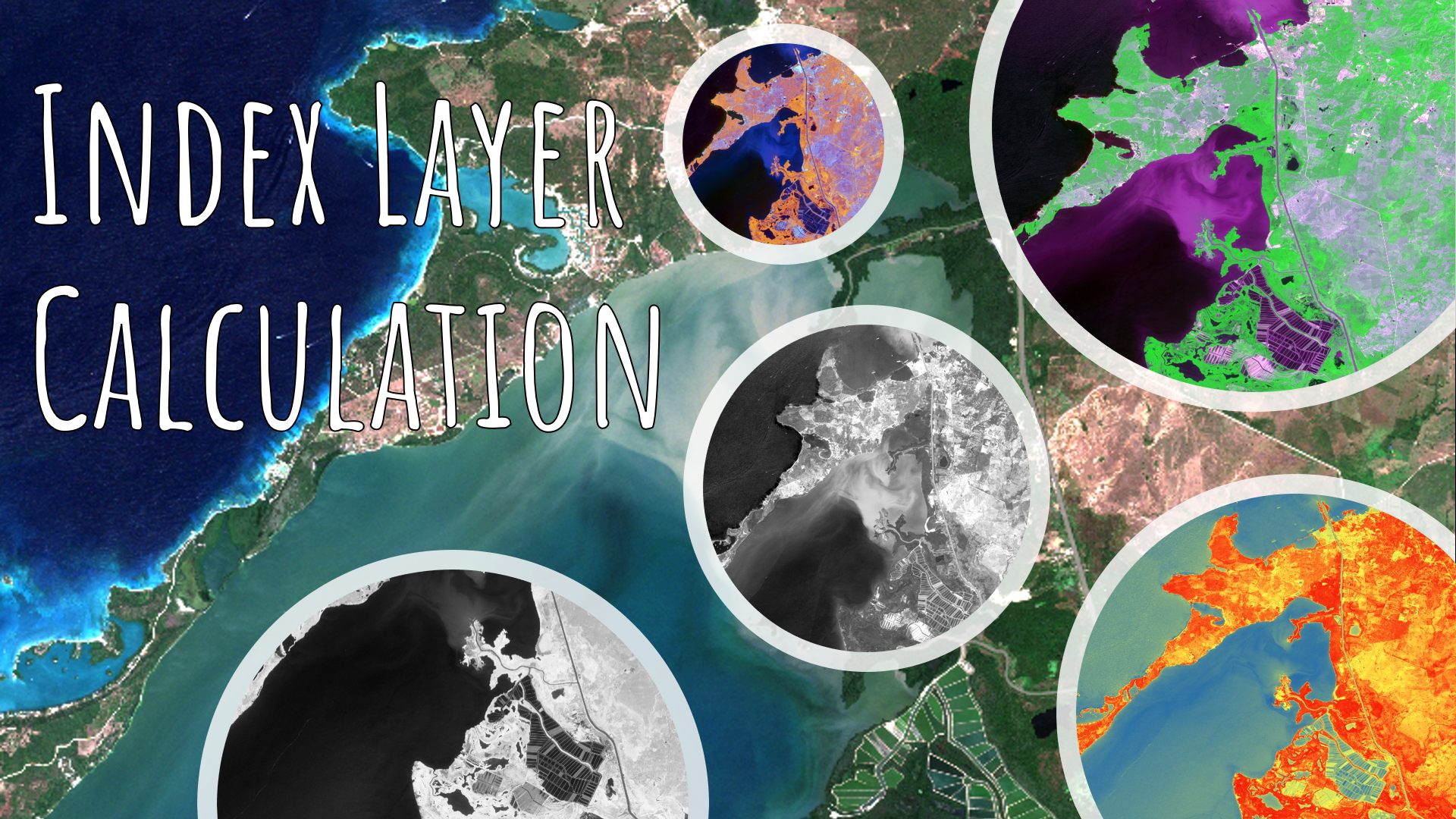We have an algorithm implemented in eCognition which enables you to directly calculate highly interesting and helpful index layers and is called "Index Layer Calculation".• NDVI - Normalized difference vegetation index - (NIR-Red)/(NIR+Red)
• NDWI - Normalized Difference Water Index - (Green-NIR)/(Green+NIR)
• NDSI - Normalized Difference Soil Index - (Red-Blue) / (Red+Blue)
• NBR - Normalized Burn Ratio - (NIR-SWIR)/(NIR+SWIR)
• SAVI - Soil Adjusted Vegetation Index - ((NIR-Red)/(NIR+Red+L))*(1+L)
• EVI - Enhanced Vegetation Index - 2.5*(Nir-Red)/(NIR+C1*Red-C2*Blue+L)
• GRVI - Green-Red Vegetation Index - (Green - Red)/(Green + Red)

Simply assign the according layers to be used for the calculation (dependent on selected index) and start creating additional layers for your project! Enjoy!